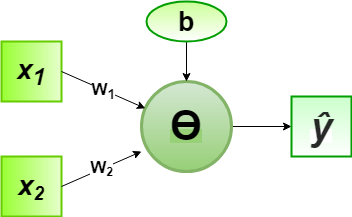Related Articles

# Implementation of Perceptron Algorithm for OR Logic Gate with 2-bit Binary Input

• Difficulty Level : Easy
• Last Updated : 08 Jun, 2020

In the field of Machine Learning, the Perceptron is a Supervised Learning Algorithm for binary classifiers. The Perceptron Model implements the following function:Attention reader! Don’t stop learning now. Get hold of all the important Machine Learning Concepts with the Machine Learning Foundation Course at a student-friendly price and become industry ready.

For a particular choice of the weight vectorand bias parameter, the model predicts outputfor the corresponding input vector.

OR logical function truth table for 2-bit binary variables, i.e, the input vectorand the corresponding output000
011
101
111

Now for the corresponding weight vectorof the input vector, the associated Perceptron Function can be defined as:For the implementation, considered weight parameters areand the bias parameter is.

Python Implementation:

 # importing Python libraryimport numpy as np  # define Unit Step Functiondef unitStep(v):    if v >= 0:        return 1    else:        return 0  # design Perceptron Modeldef perceptronModel(x, w, b):    v = np.dot(w, x) + b    y = unitStep(v)    return y  # OR Logic Function# w1 = 1, w2 = 1, b = -0.5def OR_logicFunction(x):    w = np.array([1, 1])    b = -0.5    return perceptronModel(x, w, b)  # testing the Perceptron Modeltest1 = np.array([0, 1])test2 = np.array([1, 1])test3 = np.array([0, 0])test4 = np.array([1, 0])  print("OR({}, {}) = {}".format(0, 1, OR_logicFunction(test1)))print("OR({}, {}) = {}".format(1, 1, OR_logicFunction(test2)))print("OR({}, {}) = {}".format(0, 0, OR_logicFunction(test3)))print("OR({}, {}) = {}".format(1, 0, OR_logicFunction(test4)))
Output:
OR(0, 1) = 1
OR(1, 1) = 1
OR(0, 0) = 0
OR(1, 0) = 1


Here, the model predicted output () for each of the test inputs are exactly matched with the OR logic gate conventional output () according to the truth table for 2-bit binary input.
Hence, it is verified that the perceptron algorithm for OR logic gate is correctly implemented.

My Personal Notes arrow_drop_up